# tedana.decomposition.tedpca

Use principal components analysis (PCA) to identify and remove thermal noise from multi-echo data.

Parameters
• data_cat ((S x E x T) array_like) – Input functional data

• data_oc ((S x T) array_like) – Optimally combined time series data

• combmode ({‘t2s’, ‘paid’} str) – How optimal combination of echos should be made, where ‘t2s’ indicates using the method of Posse 1999 and ‘paid’ indicates using the method of Poser 2006

• adaptive_mask ((S,) array_like) – Array where each value indicates the number of echoes with good signal for that voxel. This mask may be thresholded; for example, with values less than 3 set to 0. For more information on thresholding, see make_adaptive_mask.

• t2sG ((S,) array_like) – Map of voxel-wise T2* estimates.

• io_generator (tedana.io.OutputGenerator) – The output generation object for this workflow

• tes (list) – List of echo times associated with data_cat, in milliseconds

• algorithm ({‘kundu’, ‘kundu-stabilize’, ‘mdl’, ‘aic’, ‘kic’, float}, optional) – Method with which to select components in TEDPCA. PCA decomposition with the mdl, kic and aic options are based on a Moving Average (stationary Gaussian) process and are ordered from most to least aggressive (see Li et al., 2007). If a float is provided, then it is assumed to represent percentage of variance explained (0-1) to retain from PCA. Default is ‘aic’.

• kdaw (float, optional) – Dimensionality augmentation weight for Kappa calculations. Must be a non-negative float, or -1 (a special value). Default is 10.

• rdaw (float, optional) – Dimensionality augmentation weight for Rho calculations. Must be a non-negative float, or -1 (a special value). Default is 1.

• verbose (bool, optional) – Whether to output files from fitmodels_direct or not. Default: False

• low_mem (bool, optional) – Whether to use incremental PCA (for low-memory systems) or not. This is only compatible with the “kundu” or “kundu-stabilize” algorithms. Default: False

Returns

Notes

Notation

Meaning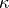Component pseudo-F statistic for TE-dependent (BOLD) model.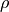Component pseudo-F statistic for TE-independent (artifact) model.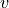Voxel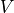Total number of voxels in mask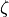Something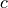Component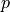Something else

Steps:

1. Variance normalize either multi-echo or optimally combined data, depending on settings.

2. Decompose normalized data using PCA or SVD.

3. Computeand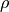:4. Some other stuff. Something about elbows.

5. Classify components as thermal noise if they meet both of the following criteria:

• Nonsignificantand.

• Nonsignificant variance explained.

Outputs:

This function writes out several files:

Default Filename

Content

desc-PCA_metrics.tsv

PCA component table

desc-PCA_metrics.json

Metadata sidecar file describing the component table

desc-PCA_mixing.tsv

PCA mixing matrix

desc-PCA_components.nii.gz

Component weight maps

desc-PCA_decomposition.json

Metadata sidecar file describing the PCA decomposition

tedana.utils.make_adaptive_mask()
The function used to create the adaptive_mask parameter.
tedana.constants